# Initial Value Theorem of Laplace TransformInitial Value Theorem is one of the basic properties of Laplace transform. It was given by prominent French Mathematical Physicist Pierre Simon Marquis De Laplace. He made crucial contributions in the area of planetary motion by applying Newton’s theory of Gravitation. His work regarding the theory of probability and statistics is considered pioneering and this influenced a whole new generation of Mathematician. Laplace is one among the 72 people to have their name engraved on the Eiffel Tower.
Initial value theorem and Final value theorem are together called as Limiting Theorems. Initial value theorem is often referred as IVT. It will enable us to find the initial value at time t = (0+) for a given transformed function (laplace) without enabling us work harder to find f(t) which is a tedious process in such case.

#### Conditions for the existence of Initial value theorem

1. The function f(t) and its derivative f(t) should be Laplace transformable.
2. If time t approaches to (0+) then the function f(t) should exists.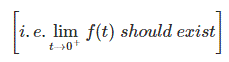1. The function f(t) = 0 for t > 0 and contains no impulses or higher order singularities at origin.

## Statement of Laplace Initial Value Theorem

If f(t) and F(s) is Laplace transform pairs. i.e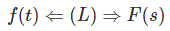then Initial value theorem is given by## Proof of Laplace Initial Value Theorem

Laplace transform of a function f(t) isthen Laplace transform of its derivative f ‘ (t) isConsider the integral part first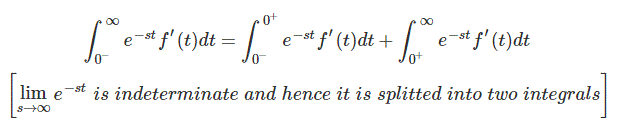Substituting (2) in (1) we get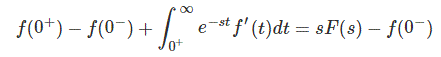Upon cancelling f (0) on both sides we get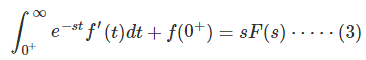We can straightaway write the above equation but my intension on taking the limits of integration from (0 to ∞) is that however we consider the negative values of limits it pertains to the results having positive values.

Note:
We also knew that Laplace transform is applicable only for causal functions.
On considering (s) tends to infinity on both sides in (3)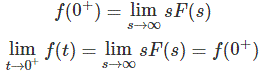Hence, Initial value theorem is proved.

### Applications of Initial Value Theorem

As I said earlier the purpose of initial value theorem is to determine the initial value of the function f (t) provided its Laplace transform is given
Example 1 :
Find the initial value for the function f (t) = 2 u (t) + 3 cost u (t)
Sol: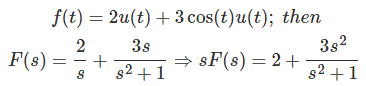By initial value theoremThe initial value is given by 5.
Example 2:
Find initial value of the transformed function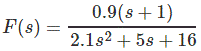Sol:By initial value theorem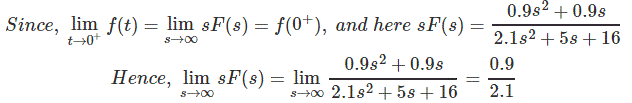[as s → ∞ the values of s become more and more insignificant hence the result is obtained by simply taking the ratio of leading co-efficient]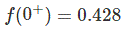Example 3:
Find the initial value ofSolution:
Initial value theorem is not applicable in this case. We can prove it in two ways.
Let’s see how it goes
Method 1:Note:
This theorem is applicable strictly if F (s) is proper fraction i.e the numerator polynomial is of lower order than the denominator polynomial.
In case if IVT is applied we get ∞ as the initial value.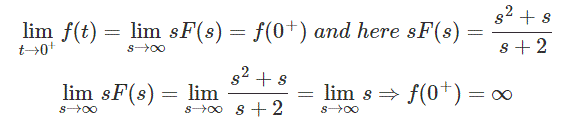[this is not possible in practical circuits ]
Aliter:Applying Inverse Laplace transform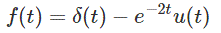It is obvious that Initial value theorem is not applicable since there is impulse function, which is constant over time t.

By this discussion, it is easy for one to manipulate the initial conditions of the circuit with the Laplace transformed function.

Want To Learn Faster? 🎓
Get electrical articles delivered to your inbox every week.
No credit card required—it’s 100% free.